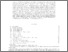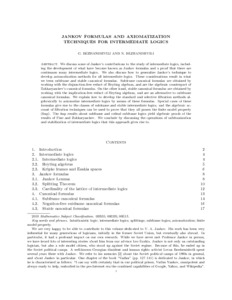# PP-2020-12: Jankov formulas and axiomatization techniques for intermediate logics

PP-2020-12: Bezhanishvili, Guram and Bezhanishvili, Nick (2020) Jankov formulas and axiomatization techniques for intermediate logics. [Pre-print]Preview Text Jankov-volume.pdf Download (437kB) | Preview

## Abstract

We discuss some of Jankov’s contributions to the study of intermediate logics, including the development of what have become known as Jankov formulas and a proof that there are
continuum many intermediate logics. We also discuss how to generalize Jankov’s technique to
develop axiomatization methods for all intermediate logics. These considerations result in what
we term subframe and stable canonical formulas. Subframe canonical formulas are obtained by
working with the disjunction-free reduct of Heyting algebras, and are the algebraic counterpart of
Zakharyaschev’s canonical formulas. On the other hand, stable canonical formulas are obtained by
working with the implication-free reduct of Heyting algebras, and are an alternative to subframe
canonical formulas. We explain how to develop the standard and selective filtration methods algebraically to axiomatize intermediate logics by means of these formulas. Special cases of these
formulas give rise to the classes of subframe and stable intermediate logics, and the algebraic account of filtration techniques can be used to prove that they all posses the finite model property
(fmp). The fmp results about subframe and cofinal subframe logics yield algebraic proofs of the
results of Fine and Zakharyaschev. We conclude by discussing the operations of subframization
and stabilization of intermediate logics that this approach gives rise to.

Item Type: Pre-print PP-2020-12 Prepublication (PP) Series 2020 LogicMathematics Nick Bezhanishvili 25 Jul 2020 18:57 25 Jul 2020 18:57 https://eprints.illc.uva.nl/id/eprint/1744View Item